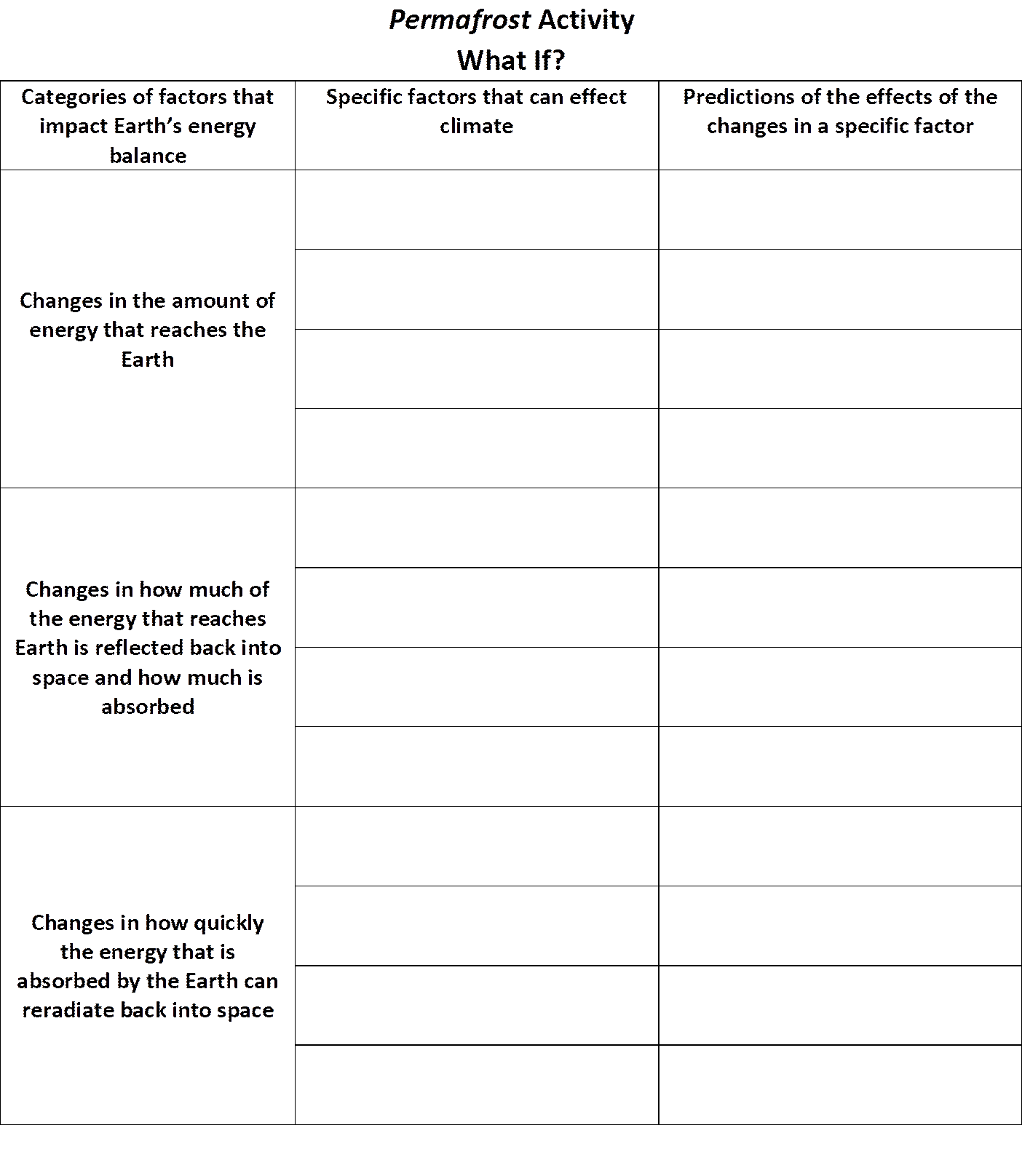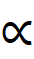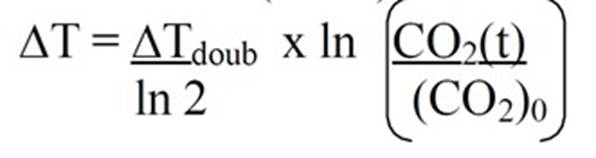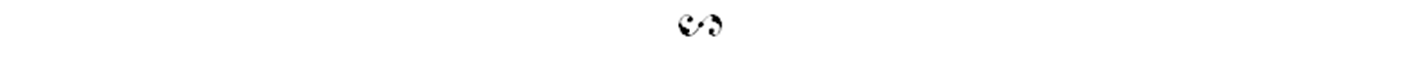Issues in Earth Science

“Eww, There’s Some Geology in my Fiction!”

Issue 11, Aug 2019

Teacher Resources

Suggestions for Activities and Discussions to accompany Readings of

Permafrost by Kate Kelly

The story in Permafrost implies a past period of global warming which caused economic and environmental stresses that led to war and the war resulted in a sharp decrease in global temperature and even more severe economic and environmental stress.  The science behind these changes in climate involve understanding how various factors affect world temperature.  This understanding is based on studies of Earth's past climate as well as numerical modeling of the inflow and outflow of energy from the Earth.  The inflow and outflow of energy from the Earth system are complex and at the frontier of modern research.  Even so, students can engage with thinking about factors and feedback loops that affect energy flow.  For example, they can think about how human activities in the past of the Permafrost world might have caused global warming and how war might have caused a subsequent descent into a global ice age.   They can also consider many other factors that affect climate, as suggested in our first activity below, a game of 'what if'' that gives students an opportunity to think about some important feedback loops.

To some extent, students can model the effect of energy flow on global temperatures quantitatively, although student quantitative predictions are necessarily simplified and uncertain.  Even climatic models by research scientists differ somewhat from each other and provide only our current best estimates of how various factors affect climate.  This uncertainty is a function of the complexity of Earth's energy balance which in turn is a consequence of complex feedback loops that are difficult to predict from basic physics principles.  Our second activity, a game of "how much?" gives students the opportunity to think about how much a particular disturbance in the global energy balance might affect global temperatures.

What if?

The goal of this activity is to encourage students to ask questions about factors that might influence Earth's climate and then answer those questions themselves through reasoning and/or online research.

The core idea in understanding changes in Earth's climate is that the energy that comes into the Earth system must also leave the Earth system, meaning that there is a balance of energy coming in and going out.  When that balance gets out of whack, that is, when energy coming in and going out are not equal, then the Earth will heat up or cool down until the balance is reinstated.  Even during times of climate change, the energy coming in and going out are very close to the same (otherwise we would see a much faster change in temperature).  Although the factors that affect this balance are complex, they can be grouped into three simple categories, the understanding of which can help students predict the qualitative effect of various changes.  Those categories are the following:

1)  Changes in the amount of energy that reaches the Earth.  For example, there might be changes in the Sun itself, or our solar system might pass near to another star, or there might be a nearby supernova which affects energy reaching the Earth.

2)  Changes in how much of that amount of energy that reaches Earth is reflected back into space without being absorbed.  Only the absorbed energy helps keep Earth warm.  The amount of Earth's energy that is reflected is called Earth's albedo.  Increases in reflective materials like ice, clouds, or reflective particles in suspension in Earth's atmosphere can increase albedo and thus cool the Earth whereas a decrease in albedo, increasing absorption, will warm the Earth.

3) Changes in how quickly the energy that is absorbed by the Earth can reradiate back into space.  All the energy that comes to the Earth eventually radiates back into space by black body radiation.  Black body radiation is the idea that all bodies emit energy at wavelengths and in amounts that depend on their temperature.  Because of its temperature, Earth is "glowing" in infrared, a wavelength of light that our eye cannot see.  Greenhouse gases are gases that absorb infrared light, but not visible light, and thus delay the reradiated energy's journey back into space, keeping the energy in the Earth system longer and making the Earth warmer.

Encourage students to ask "what if" questions that address how a particular disturbance to Earth's energy balance might affect global temperatures.  By considering the three categories above, students can predict the effect of a large number of factors on Earth's climate.  For example, students might ask "what if the amount of ice at the poles decreases?"  They might either realize that ice is reflective, meaning that decreasing the ice will decrease albedo and warm the planet, or they can search online using key words such as "what is the affect of ice on albedo?"  Make a game out of it:  How many different factors can they identify that 1) have an effect on climate and 2) whose effect they can correctly predict?

Although the goal of this activity is for students to come up with, and answer, their own questions, offering an example "what if" question, like the one above, can help get them started.  Another way to help them come up with questions is to provide a structure for thinking about factors that might affect the Earth's energy balance, such as the table below.Students might come up with some factors and what if questions that are difficult or impossible for them to answer (for example,  What if Earth's magnetic field switched polarity?).  However, some questions students might come up with and reasonably answer with their own reasoning and resources they find on line include the following.

What if increasing temperature melts polar ice?

What if increasing temperature raises ocean temperatures? (think about how CO2 dissolves or undissolves from water at different temperatures)?

What if increasing temperature allows plant growth farther north (think about the effect that plant growth has on CO2)?

What if increasing temperatures melts arctic permafrost (think about carbon that is frozen into the permafrost)?

What if dust or aerosols from volcanic eruptions increase the amount of sunlight reflected from Earth?

What if dust or aerosols from nuclear war increase the amount of sunlight reflected from Earth?

What if soot from manufacturing processes decrease the amount of sunlight reflected from the Earth (not all particles in the atmosphere are reflective)?

What if increasing temperatures increases the amount of water vapor (a greenhouse gas) in the air (more water vapor can be in warmer air)?

What if increasing water vapor in the air causes an increase in reflective clouds (if there is more water vapor due to higher temperatures, there is the potential for more clouds, although this is complex and one of the hardest things for climate models to predict)?

How Much?

The goal of this activity is to give students a deeper quantitative understanding of the energy balance that controls Earth's climate and to provide the opportunity to practice mathematical thinking.

As mentioned above, numerical modeling of climate change is complex and an area of ongoing research.  However, students can gain an understanding of how numerical modeling works through some simple numerical predictions based on two relationships.

Part 1:  Relationship between albedo and temperature

The theoretical relationship relates Earth's temperature to how much energy we can expect it to emit by black body radiation.

Energy emitted by blackbody radiation is proportional to temperature to the fourth power (T4).  This means that the temperature is related to energy by the expression

T(energy emitted)1/4.

If we increase the amount of energy emitted by 1%, the temperature will increase to 1.011/4 of its previous value (=1.0025).  If it started out at 0°C (273K) then we might expect an increase to about 273K x 1.0025 = 273.6K, or about 0.6K (0.6°C increase or a little over 1 degree Fahrenheit).  Remembering that the amount of energy emitted by the Earth will be roughly in balance with the amount of energy absorbed by the Earth, we can figure out changes in the amount of energy emitted by considering changes in how much of the Sun's energy gets reflected instead of absorbed.

Students can ask questions about how changes in Earth's albedo might affect world temperature.  Right now, on average, about 31% of Earth's sunlight gets reflected, leaving 69% to be absorbed.  How will changes in the albedo affect world temperature?

For example, suppose that ice at the poles melted, causing the amount reflected to decrease to 29%.  How much of a change in temperature do you expect? (hint:  this will increase the energy absorbed by about 2.9% as calculated from [71%-69%]/69% x 100%.  You can use the same reasoning and method as in the calculated example above to figure out how big a temperature change to expect.).

Suppose that dust and aerosols injected into Earth's stratosphere by a nuclear war causes Earth's albedo to increase to 35%.  How much of a change in temperature do you expect?

Suppose that the dust and aerosols cause average cloud cover on the Earth to increase (due to cooler temperatures and/or more nuclei on which water droplets might condense), causing albedo to increase further to 37%.  How much of a change in temperature do you expect?

Part 2:  Relationship between carbon dioxide and temperature

Due to the complex factors influencing the relationship between greenhouse gases and temperature, a purely theoretical prediction is not possible at the present time, however, we can look at how CO2 and global temperature have covaried in the geological past (for example over the past 10000 years and over the longer period of time since the time of the dinosaurs.)

One expression that is used to model the effect of CO2 on global temperature is the following, where DT is the change in global temperature at some future time t after time 0, DTdoub  is the change in temperature that will result if the CO2 concentration in the atmosphere doubles,  (CO2)0 is the concentration of CO2 at some time 0, and CO2(t) is the concentration of CO2 at some future time t after time 0.  ln is the natural log function.This expression predicts that temperature will increase as the natural log of concentration of CO2 increases.  The expression depends on the parameter DTdoub , an empirical value which is too complex to predict theoretically must be determined by observation.  An estimate of this value can be determined by looking at how global temperature and CO2 have changed in the geological past.  Estimated values often lie between 1.5 and 4.5°C, based on past correlations between CO2 concentrations and global temperature.  However, in applying this empirical relationship, students should be aware of the difference between correlation and causation.  Although we can see the correlation between temperature and CO2 concentrations in high-quality data such as ice cores, these data alone cannot prove that the CO2 increase is causing the increase in temperature.  Thus, there is some uncertainty in how much variation in temperature can be expected based on a given change in CO2—it might not be the same in the future as it has been in the past.

An activity which allows students to make their own empirical estimates of DTdoub can be found at https://archive.epa.gov/climatechange/kids/documents/temp-and-co2.pdf.  Students will need some additional prompting from the teacher to plot temperature vs CO2 which is not done in the exercise because it implies a causative relationship which cannot be confirmed by the data shown.

Given the relationship in the mathematical expression above, students can predict the effects of increasing CO2 on global temperatures.  If CO2 in the atmosphere continues to increase exponentially as it has over the past 100 years or so, then we might expect the CO2 to have doubled by 2100.  How much change in temperature would we expect?  (choosing an intermediate DTdoub = 3, DT = [3 ÷ln2] x ln2 = 3°C.)

What if it doubled again in another hundred years? (choosing an intermediate DTdoub = 3, DT = 3 ÷ln2 x ln4 = 6°C.)

Part3:  Combining changes in albedo with changes in greenhouse gases

Given the two mathematical models above, students can answer more complex questions that combine the two mathematical relationships.  For example, in the story Permafrost, how much of an increase in Earth's albedo is required to overcome the global warming effects due to greenhouse gases that was already ongoing?  Rather than giving your students the numbers to plug into the equations—which doesn't really give them the sense of either a science investigation or of mathematical thinking—encourage your students to figure out reasonable numbers to use.  How much of a change in temperature would count as a global warming event?  How much of a drop in temperature would be required to produce a global ice age?  These are estimates students can make using information that is available online and from that information they can make decisions about numbers to use in the equations.  Take note that students are not simply "looking up information," rather they are using online information to allow them to make informed judgements about a related problem.

Resources

These activities are research activities for the students, and, ideally, will involve the teacher learning new things as well.  However, as background for the teacher, some introductory ideas about global energy balance can be found in Russ's self-guided online course notes at

http://web.mnstate.edu/colson/ESE/energy_balance_of_worlds.html

Some examples of energy-balance feedback loops can be found at

http://web.mnstate.edu/colson/ESE/greenhouse_warming.html

Connecting to the Next Generation Science Standards.

Students can exercise skills in the practices of science including:

1) Asking Questions—in the What If? activity, students ask questions about Earth’s energy balance and changes that might occur within the Earth system. The student questions arise from their observations of nature, from their examination of models, or from their need to seek additional information.

and

2) Using Mathematics—in the How Much? activity, students use mathematical expressions to describe the relationships between different climatic variables (like temperature and energy or carbon dioxide concentration and temperature).

Students use the crosscutting concepts of

1) Systems and System Models—in both activities as they consider inputs and outputs and interactions within and among systems

2) Stability and Change—in the How Much? activity as students quantify change and rates of change of periods of time; in the What If? activity as students consider the stability of Earth’s energy balance and how that balance might be disturbed over time.

The investigative activities above support the following NGSS performance expectations:

HS-ESS2-2. Analyze geoscience data to make the claim that one change to Earth's surface can create feedbacks that cause changes to other Earth systems.

HS-ESS2-4. Use a model to describe how variations in the flow of energy into and out of Earth’s systems result in changes in climate.

HS-ESS3-5. Analyze geoscience data and the results from global climate models to make an evidence-based forecast of the current rate of global or regional climate change and associated future impacts to Earth's systems.———————————————————————————

The Teacher Resources for Permafrost are written by Russ and Mary Colson, authors of Learning to Read the Earth and Sky.

Return to  Permafrost by Kate Kelly

Return to “Eww, There’s Some Geology in My Fiction.”

Find more essays, games, and stories at

©2019 Issues in Earth Science